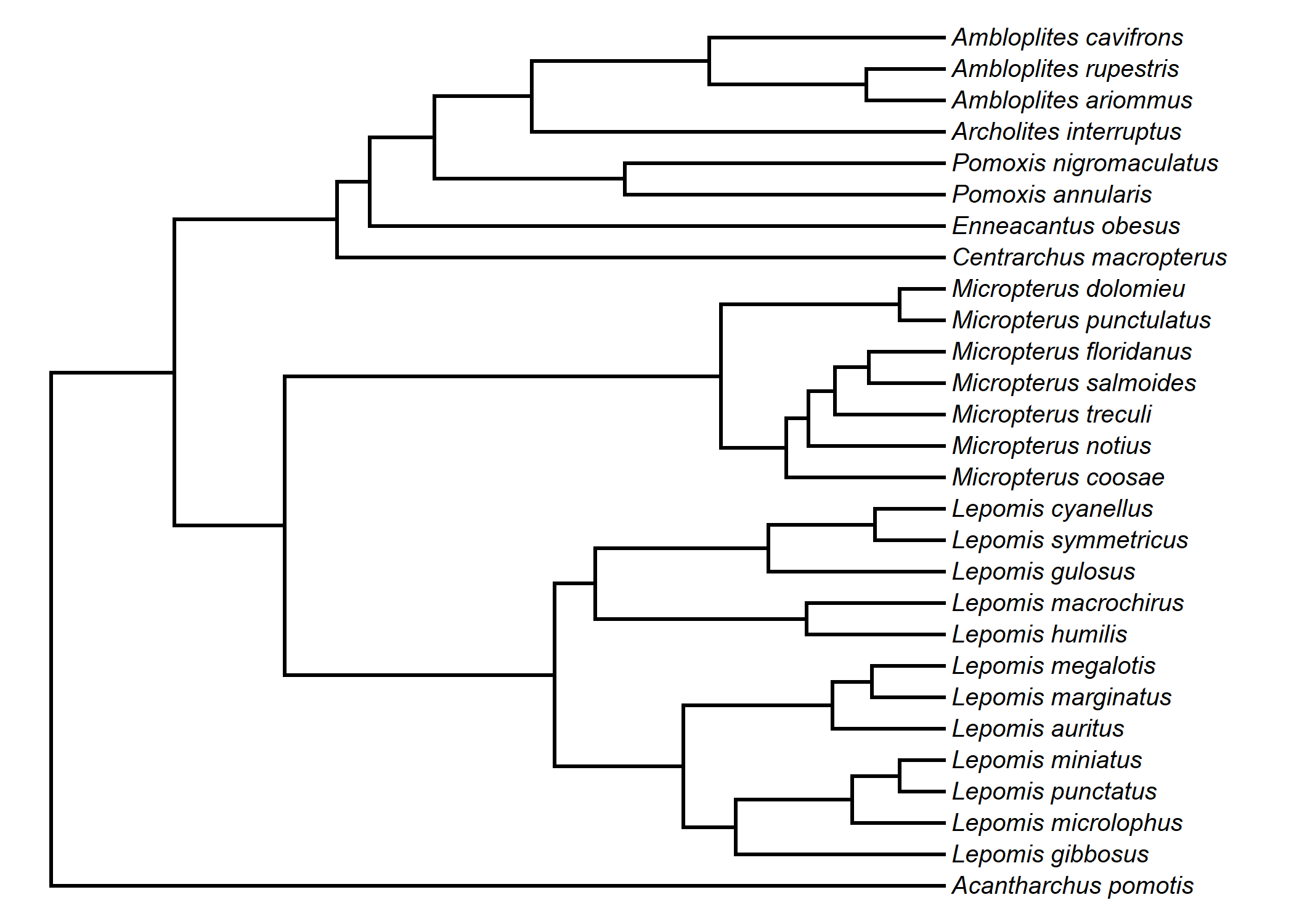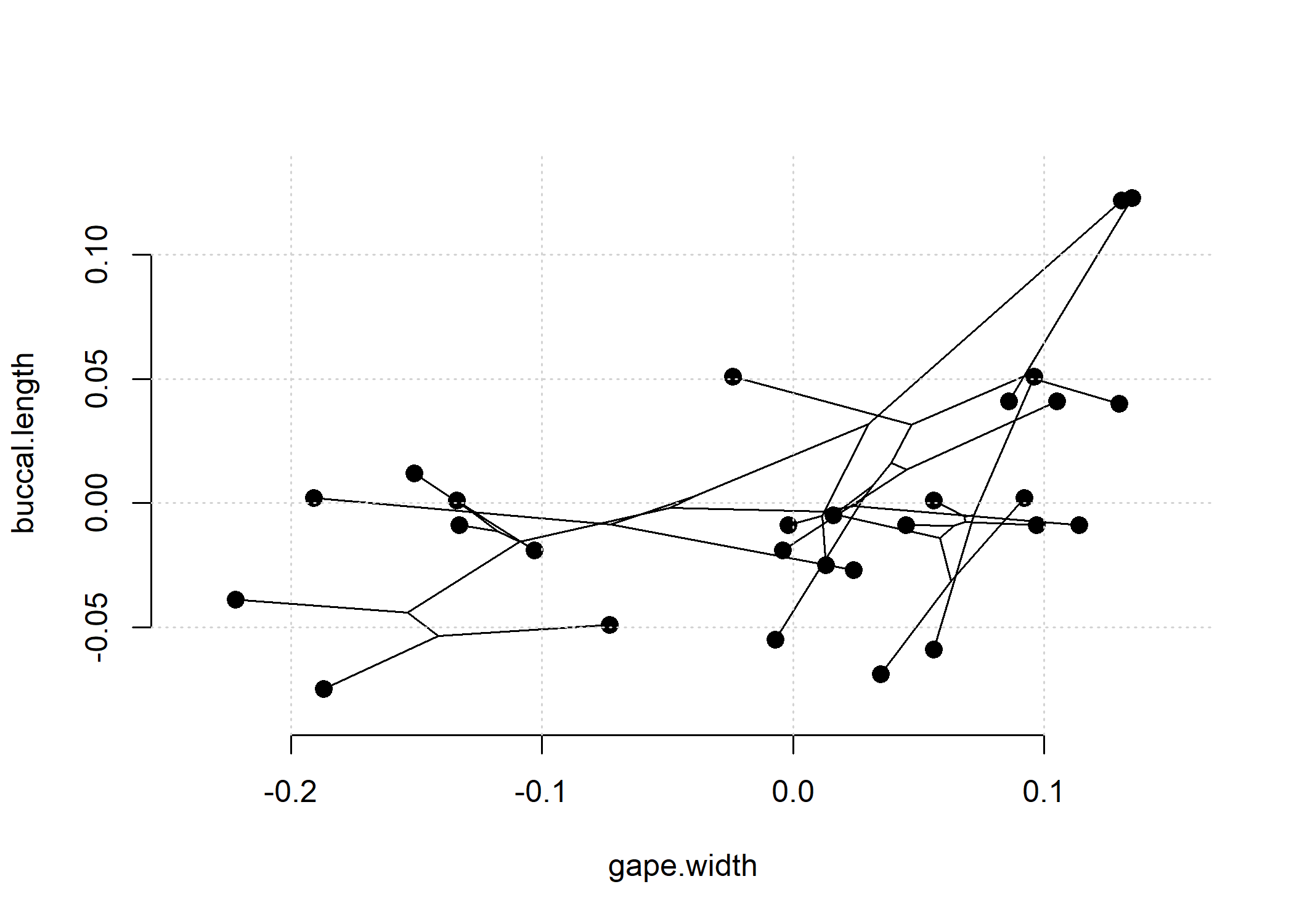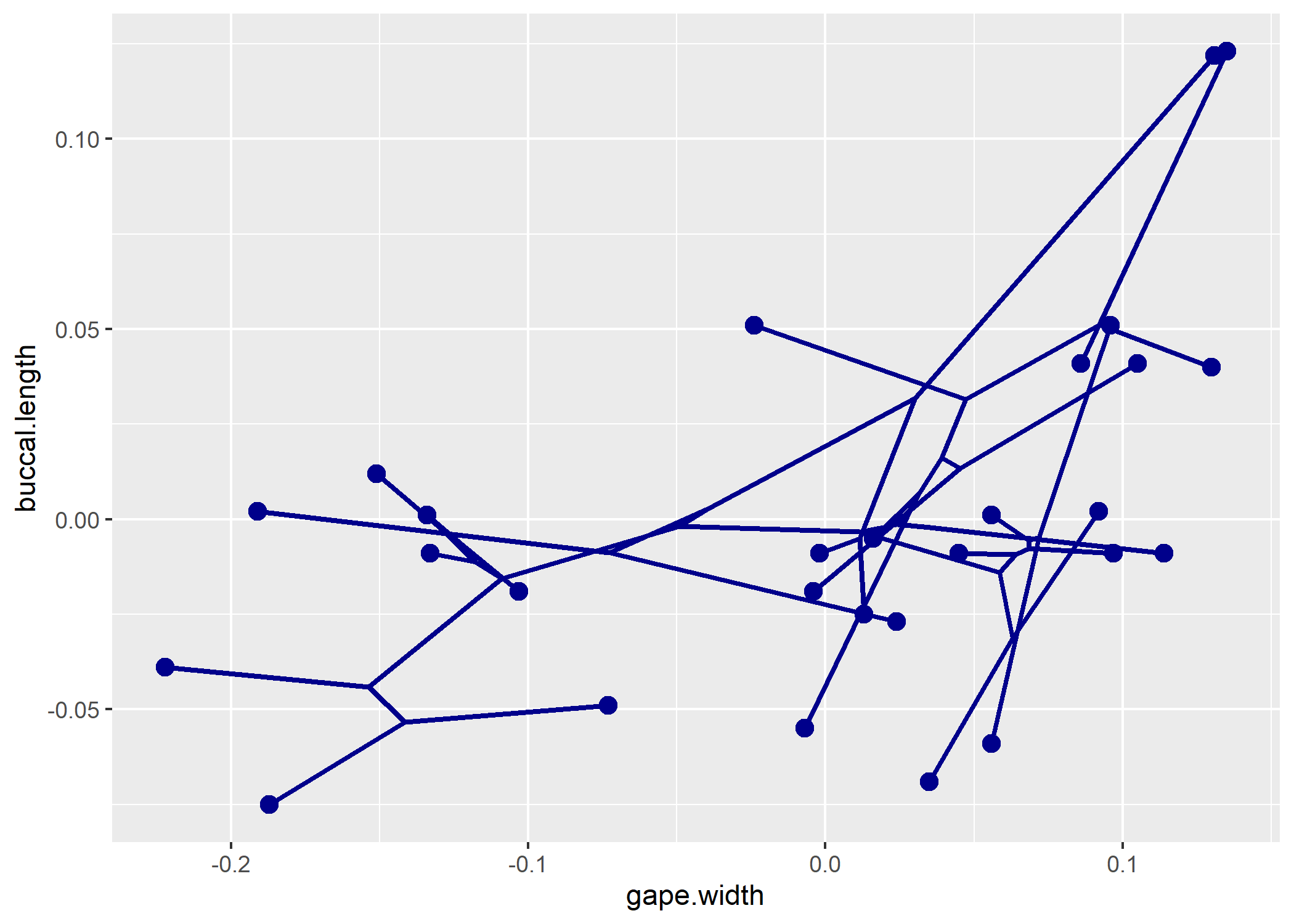## Wednesday, December 14, 2022

### Generating a ggplot phylomorphospace plot with phytools

Today a colleague & phytools user, Werner de Gier, tweeted the following about using phytools to graph a phylomorphospace projection with ggplot2.

My reaction was the following.

I nonetheless promised to share his solution on this blog – assuming that I could get it to work without the Microsoft Excel step!

Here it is.

``````library(phytools)
library(ggplot2)
``````

Now let’s pull in our data.

These come from Revell & Collar (2009).

``````data(sunfish.tree)
tree<-as.phylo(sunfish.tree)
plotTree(tree,ftype="i",fsize=0.8)
````````````data(sunfish.data)
``````
``````##                      feeding.mode gape.width buccal.length
## Acantharchus_pomotis         pisc      0.114        -0.009
## Lepomis_gibbosus              non     -0.133        -0.009
## Lepomis_microlophus           non     -0.151         0.012
## Lepomis_punctatus             non     -0.103        -0.019
## Lepomis_miniatus              non     -0.134         0.001
## Lepomis_auritus               non     -0.222        -0.039
``````
``````X<-sunfish.data[,2:3]
``````

Now let’s create a phylomorphospace projection using phytools.

``````obj<-phylomorphospace(tree,X,ftype="off",bty="n",node.size=c(0,1.2))
grid()
``````As Werner pointed out, `phylomorphospace` returns the phylomorphospace coordinates to the user invisibly. Let’s use them to create a data frame to be passed to `ggplot2::geom_segment`.

``````Data<-data.frame(
xstart=obj\$xx[obj\$edge[,1]],
ystart=obj\$yy[obj\$edge[,1]],
xstop=obj\$xx[obj\$edge[,2]],
ystop=obj\$yy[obj\$edge[,2]],
nodestart=obj\$edge[,1],
nodestop=obj\$edge[,2])
``````

Here’s what that looks like:

``````head(Data)
``````
``````##         xstart       ystart       xstop        ystop nodestart nodestop
## 1   0.03520284 -0.002225660  0.11400000 -0.009000000        29        1
## 30  0.03520284 -0.002225660  0.02429463 -0.001287860        29       30
## 31  0.02429463 -0.001287860  0.01151996 -0.003347729        30       31
## 32  0.01151996 -0.003347729 -0.04908695 -0.001822143        31       32
## 33 -0.04908695 -0.001822143 -0.10899316 -0.015630236        32       33
## 34 -0.10899316 -0.015630236 -0.11776881 -0.011263323        33       34
``````

Finally, let’s create out plot!

``````ggplot()+
geom_point(data= X,aes(x=gape.width,y=buccal.length),
size=3,color="darkblue")+
geom_segment(data=Data,aes(x=xstart,y=ystart,xend=xstop,yend=ystop),
size = 1,color="darkblue")
``````Neat. We’re done.